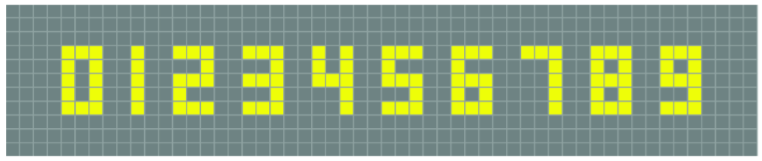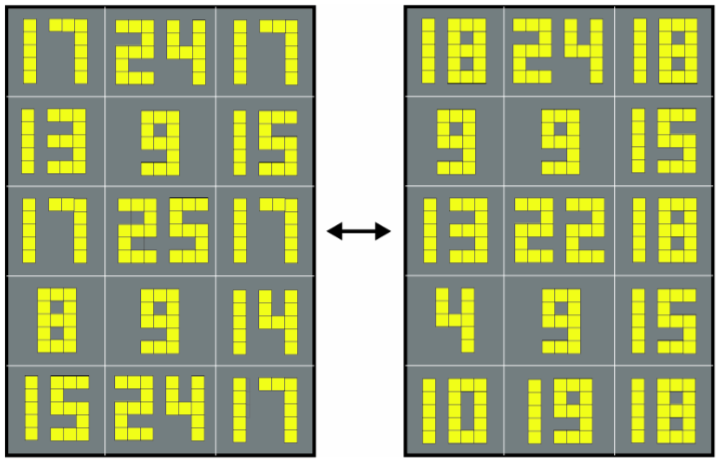From Lee Sallows:

Earlier this year, Futility Closet featured a puzzle based upon the well-known 7-segment display. Less well known is the 15-cell display shown in Figure 1, in which each decimal digit appears as a pattern of highlighted cells within a 3×5 rectangle. Call these the small rectangles. Observe also that the digit 1 is represented as the vertical column of 5 cells in the centre of its small rectangle rather than as either of the two alternative columns immediately to left and right, a detail that is important in view of what follows.Figure 1.

Figure 2 shows a pair of readouts using 15-cell displays each arranged in the form of a (large) 3×5 rectangle that mirrors the smaller rectangles just mentioned. The two readouts describe each other. The top left cell in the right-hand readout contains the number 18. A check will show that the number of highlighted top left cells appearing in the left-hand readout is indeed 18. Take for instance the top left-hand cell in the left-hand readout. It contains the number 17, which employs two digits, 1 and 7. None of the 5 cells forming the digit 1 is in top left position within its small rectangle. But the leftmost cell in digit 7 is indeed in top left position. Proceeding next to the left-hand readout’s top centre cell we find two cells in top left position: one in digit 2 and one in digit 4. The score of top left cells so far is thus 1+2 = 3. Continuing in normal reading order, a list of the left-hand readout numbers followed by their top left cell scores in brackets is as follows: 17 (1), 24 (2), 17(1), 13(1), 9(1), 15(1), 17(1), 25(2), 17(1), 8(1), 9(1), 14(1), 15(1), 24(2), 17(1). The sum of the scores is 18, as predicted.

In the same way, a number occupying position x in either of the readouts will be found to identify the total number of cells occurring in position x within the digits of the other readout. That is, the two readouts are co-descriptive, they describe each other.Figure 2.

Recalling now the solution to the earlier mentioned 7-segment display puzzle, some readers may recall that it involved an iterative process that terminated in a loop of length 4. Likewise, the pair of readouts in Figure 2 are the result of a similar process, but now terminating in a loop of length 2. In that case we were counting segments, here we are counting cells. An obvious question thus prompted is: What kind of a readout would result from a loop of length 1? The answer is simple: a description of the readout resulting from a loop of length 1 would be a copy of the same readout. That is, it will be a self-descriptive readout, the description of which is identical to itself. Such a readout does indeed exist. Can the reader find it?